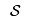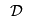Difference: TransportationProblemInAMPL (3 vs. 4)

Revision 42008-04-02 - MichaelOSullivan

Line: 1 to 1

 META TOPICPARENT name="AMPLGuide"

The Transportation Problem in AMPL

AMPL Formulation

Changed:
<
<
The formulation of the transportation problem is AMPL is a straighforward translation of the matehmatical programme for the transportation problem.
>
>
The formulation of the transportation problem is AMPL is a straightforward translation of the mathematical programme for the transportation problem.
The setsandare declared as SUPPLY_NODES and DEMAND_NODES respectively:

Line: 55 to 55

Balancing Transportation Problems

>
>
Balanced transportation models are preferred as there is no confusion about the relational operators for the supply and demand constraints. We can use script file to balance any transportation problem automatically:
reset;

model transportation.mod;

param costFromDummy {DEMAND_NODES} default 0;
param costToDummy   {SUPPLY_NODES} default 0;

param difference;

# Add the problem date file here
# e.g., data brewery.dat;

let difference := (sum {s in SUPPLY_NODES} Supply[s])
- (sum {d in DEMAND_NODES} Demand[d]);

if difference > 0 then
{
let DEMAND_NODES := DEMAND_NODES union {'Dummy'};
let Demand['Dummy'] := difference;
let {s in SUPPLY_NODES} Cost[s, 'Dummy'] := costToDummy[s];
}
else if difference < 0 then
{
let SUPPLY_NODES := SUPPLY_NODES union {'Dummy'};
let Supply['Dummy'] := - difference;
let {d in DEMAND_NODES} Cost['Dummy', d] := costFromDummy[d];
}; # else the problem is balanced

# Make sure the problem is balanced
check : sum {s in SUPPLY_NODES} Supply[s] = sum {d in DEMAND_NODES} Demand[d];

option solver cplex;

solve;

display Flow;
Note the check statement to ensure that the balancing has been done properly before solving. Also, note that costToDummy and costFromDummy allow for the definition of costs on any flow from/to a dummy node in the data file.

Both the AMPL model file transportation.mod and script file transportation.run are attached.

-- MichaelOSullivan - 02 Apr 2008

 META FILEATTACHMENT attachment="latex526e21edb215dffbfdb5ee74dd06358b.png" attr="h" comment="" date="1207132191" name="latex526e21edb215dffbfdb5ee74dd06358b.png" stream="GLOB(0xa44d1b8)" tmpFilename="latex526e21edb215dffbfdb5ee74dd06358b.png" user="MichaelOSullivan" version="1"
Line: 62 to 106

 META FILEATTACHMENT attachment="latexa518db3a714367f452620a2d18e0d61e.png" attr="h" comment="" date="1207132191" name="latexa518db3a714367f452620a2d18e0d61e.png" stream="GLOB(0xa4568f8)" tmpFilename="latexa518db3a714367f452620a2d18e0d61e.png" user="MichaelOSullivan" version="1" attachment="latex57f16e685545faa46af73ecce6b4eb7c.png" attr="h" comment="" date="1207132191" name="latex57f16e685545faa46af73ecce6b4eb7c.png" stream="GLOB(0xa44d5e4)" tmpFilename="latex57f16e685545faa46af73ecce6b4eb7c.png" user="MichaelOSullivan" version="1" attachment="latex15b62f2a4b15b2f0ea551529e221b54f.png" attr="h" comment="" date="1207132191" name="latex15b62f2a4b15b2f0ea551529e221b54f.png" stream="GLOB(0xa44d950)" tmpFilename="latex15b62f2a4b15b2f0ea551529e221b54f.png" user="MichaelOSullivan" version="1"